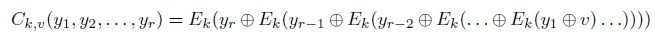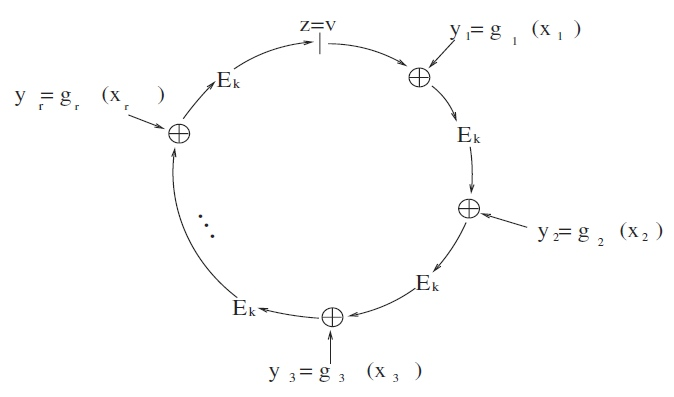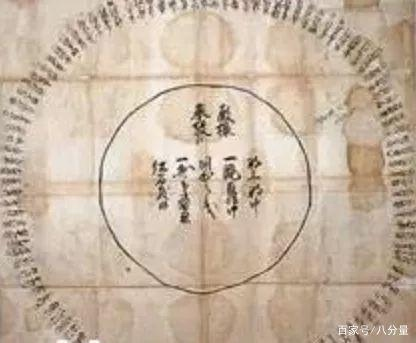## 环签名（ring Signature）

1.无条件匿名性: 攻击者者无法确定签名是由群组中哪个成员生成,即使在获得环成员私钥的情况下,概率也不超过1/r【r是群组中成员数量】。

2.不可伪造性: 群组中其他成员不能伪造真实签名者签名,攻击者者即使在获得某个有效环签名的基础上,也不能为消息m伪造一个签名。

## 环签名流程

1. 令k = hash ( m )，k作为对称加密函数E的密钥
2. 选择随机值v
3. 随机选取r-1个值 \${x_1,x_2,x_4,...x_r}\$, 并计算\$y_i=g_i(x_i)\$，计算得到相应的\${y_1,y_2,...,y_r} \$（除了\$y_j\$）
4. 令\$C_{k,v}=(y_1,y_2,...,y_r)=v\$ ， 计算出\$y_j\$
5. \$y_j\$公钥加密得到，利用私钥反向计算\$x_j=g_j^1(y_j)\$
6. 组合消息m的环签名，是一个2r + 1元组\$（P_1,P_2,...,P_r;v;x_1,x_2,...x_r）\$ ，

1. 通过公钥\$P_1,...,P_r\$, 计算\$y_i=g_i(x_i)\$, 加密得到\$y_1,...,y_r\$
2. 计算 k = Hash ( M ),
3. 验证等式 \$C_{k,v}(y_1,y_2,...,y_r)=v\$ 是否成立

## RSA环签名

1. 选择对称密钥：k = hash(m)；
2. 随机均匀选择初始值v；
3. 签名者为其他环成员均匀随机\$x_i\$，并计算\$y_i=g_i(x_i)\$；函数gi单向陷门函数，可令\$g_i(x)=x^{e_i}\ mod\ n\$
4. 根据组合函数C(k,v)的公式, 计算自己的\$y_{j'}\$, 其中\$E_k(m) = m\ xor\ k\$5.签名者利用私钥求解\$x_j=g_j^1(y_j)\$ ；6.得到消息m上的签名为\$（P_1,P_2,...,P_r;v;x_1,x_2,...x_r）\$ ；

## 小结

BTW，关于环签名还有一个有趣的历史故事，最早可以追溯到十七世纪的法国。相传，法国群臣向国王提意见的时候，为了不让国王查出是谁带的头，便采用了这种环形签名的方式，所有人的姓名以圆环的形式排列，隐匿了顺序，首倡人也就无从查起。（图片来源网络）

### 相关阅读

Schorr 签名基础篇 Schorr签名与椭圆曲线

• 发表于 2021-05-31 09:53
• 阅读 ( 3402 )
• 学分 ( 8 )
• 分类：入门/理论